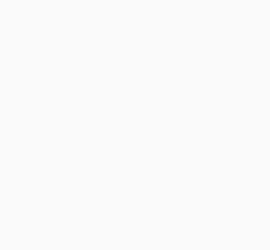# geometry tricks for ssc

1 post## GEOMETRY AND MENSURATION

GEOMETRY MENSURATION QUANTITATIVE APTITUDE Acute angle: The angle which is less than 900 Right angle: The angle which is equal to 900 Obtuse angle: The angle which is greater than 900 Straight angle: The angle which is 1800 Reflexive angle: The angle which is between 1800 to 3600 Complete angle: […]